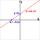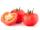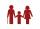# Basic functions - math word problems

1. Repair pipe20 workers had to repair broken pipes in 30 days. After fourteen days, four laborers joined them. How long did the pipe repair work last?
2. GirlsThe boys and girls in the class formed without the rest of the fives, 2 girls and 3 boys. There are 6 girls missing to create mixed pairs (1 boy and 1 girl). How many girls are in the classroom?The angle of a straight road is approximately 12 degrees. Determine the percentage of this road.
4. Inscribed sphereHow many percents of the cube volume takes the sphere inscribed into it?
5. SnacksThe school attends 344 pupils. Half of them take snacks. 13 pupils who took snacks did not attend school. How many snacks left?
6. TaxiA taxi ride costs \$3 plus \$2.50 per mile. Write and Graph an equation in two variables that represents the total cost of a taxi ride.
7. Enlarged rectangleThe rectangle with dimensions of 8 cm and 12 cm is enlarged in a ratio of 5:4. What are the circumference and the area of the enlarged rectangle?
8. Cancel fractionsCompress the expression of factorial: (n+6)!/(n+4)!-n!/(n-2)!
9. HusseinHussein owns 450000. He spent at the bookstore 2 over 9 to buy some books and tales. He paid 3 over 5 of his money to buy his math book. Then he paid his money to buy stories diagram ; a. Calculate the remaining amount of money with Hussein? b. Hussein
10. Candy and boxesWe have some number of candy and empty boxes. When we put candies in boxes of ten, there will be 2 candies and 8 empty boxes left, when of eight, there will be 6 candies and 3 boxes left. How many candy and empty boxes left when we put candies in boxes of
11. Two gardenersThe garden with an area of 81 square meters was divided by two gardeners in a ratio of 4: 5. How much did the second gardener get more first?
12. The math testThe math test contains 20 problems. For each correctly solved problem, the solver gets 3 points, for each incorrectly solved or unsolved problem, 2 points are deducted. Ondrej got 25 points. How many problems did he solve correctly?
13. Farmers 2On Wednesday the farmers at the Grant Farm picked 2 barrels of tomatoes. Thursday, the farmers picked 1/2 as many tomatoes as on Wednesday. How many barrels of tomatoes did the farmers pick on Thursday?
14. SchoolLess than 500 pupils attend school. When it is sorted into pairs, one pupil remains. Similarly, when sorted into 3, 4, 5 and 6 members team one remains. Sorted to seven members teams, no left behind. How many pupils are attending this school?
15. Ratio of sides 2The ratio of the side lengths of one square to another is 1:2. Find the ratio of the area of the two squares.
16. MerchantThe merchant lower cost by 10% in December when it was not sold. Again in January lower cost by 20% and now costs 576 €. A. How much the goods stood originally? B, how much cost the goods after the first lowering? C, How many percents totally merchant l
17. Paul earnedPaul earned 300 Kč in one hour, Václav 1/3 more than Paul. Václav worked 60 hours, which is 1/3 fewer hours than Paul worked. How many percents less earned Paul an hour than Václav? How many hours did Paul more than Václav? How much did Paul earn more
18. Young mathematicianOne young mathematician was bored again. He found that the average age of people in the room where the seminar is equal to its count. Then his 29-year-old brother entered this room. Even then, the average age of all present was the same as the count of pe
19. Ratio of sidesThe triangle has a circumference of 21 cm and the length of its sides is in a ratio of 6: 5: 3. Find the length of the longest side of the triangle in cm.
20. Solutions, mixturesHow many liters of 70% solution we must add to 5 liters of 30% solution to give us a 60% solution?

Do you have an interesting mathematical word problem that you can't solve it? Submit math problem, and we can try to solve it.

We will send a solution to your e-mail address. Solved examples are also published here. Please enter the e-mail correctly and check whether you don't have a full mailbox.

Please do not submit problems from current active competitions such as Mathematical Olympiad, correspondence seminars etc...